# Electronics and Communication Engineering - Materials and Components

### Exercise :: Materials and Components - Section 4

21.

Semiconductors have a positive temperature coefficient of resistivity.

 A. True B. False

Explanation:

In semiconductors the temperature coefficient of resistivity is negative.

22.

From a homogeneous and isotropic dielectric material of dielectric constant ∈r, a small volume element is taken out creating a cavity. The field can be kept homogeneous if Di. (i.e., electric flux density inside the cavity) and D0 (i.e., electric flux density outside the cavity) are such that

 A. D0 = Di B.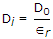C.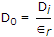D. either (a) or (b)

Explanation:

Since the field is homogeneous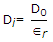.

23.

An electrolytic capacitor can be used for

 A. ac only B. dc only C. both ac and dc D. high frequency ac

Explanation:

No answer description available for this question. Let us discuss.

24.

Figure shows a composite capacitor. If CA, CB, CC are the capacitances of capacitors A, B, C, the overall capacitance C is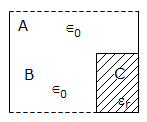A. C = CA + CB + CC B. C = (CA + CB) CC C. C =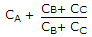D. C =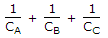Explanation:

B and C are in series and A is in parallel with this combination.

25.

As regards ferromagnetic Curie temperature Qf and paramagnetic curie temperature θ

 A. Qf > θ B. θ > Qf C. θ equal to or less than Qf D. θ may be equal to less than or more Qf